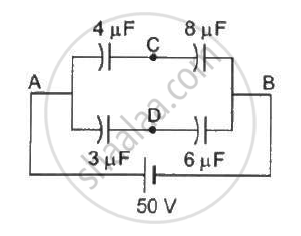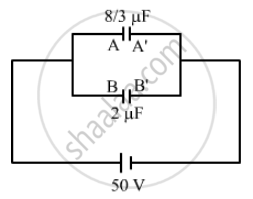Department of Pre-University Education, KarnatakaPUC Karnataka Science Class 12
Share

# Take the Potential of the Point B in Figure to Be Zero. (A) Find the Potentials at the Points C and D. (B) If a Capacitor is Connected Between C and D, What Charge Will Appear on this Capacitor? - Physics

ConceptPotential Due to a Point Charge

#### Question

Take the potential of the point B in figure to be zero. (a) Find the potentials at the points C and D. (b) If a capacitor is connected between C and D, what charge will appear on this capacitor?#### Solution(a) The capacitance of the two rows connected in parallel is given by

C_1 = (4 xx 8)/(4+8) = 8/3  "uF" and C_2 = (3 xx 6)/(3+6) = 18/9 "uF" = 2  "uF"

As the two rows are in parallel, the potential difference across each row is the same and is equal to 50 V.

The charge on the branch ACB with capacitance 8/3 "uF" is given by

Q = (8/3  "uF") xx (50 V) = 400/3 "uC"

The charge on the branch ADB with capacitance 2  "uF" is given by

Q = C xx V

Q = 2  "uF" xx 50 = 100  "uC"

The potential at point D is given by

V_D = q/C_1 = (100  "uC")/(6  "uF")

V_D = 50/3 V

Similarly, the potential at point C is given by

V_c = 50/3 V

(b) As the potential difference between points C and D is zero, the bridge remains balanced and no charge flows from C to D. If a capacitor is connected between points C and D, then the change on the capacitor will be zero.

Is there an error in this question or solution?

#### Video TutorialsVIEW ALL 

Solution Take the Potential of the Point B in Figure to Be Zero. (A) Find the Potentials at the Points C and D. (B) If a Capacitor is Connected Between C and D, What Charge Will Appear on this Capacitor? Concept: Potential Due to a Point Charge.
S